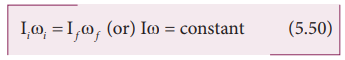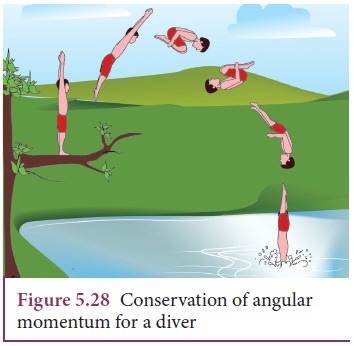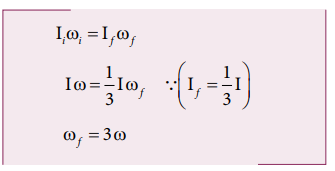Home | | Physics 11th std | Conservation of Angular Momentum

# Conservation of Angular Momentum

When no external torque acts on the body, the net angular momentum of a rotating rigid body remains constant.

Conservation of Angular Momentum

When no external torque acts on the body, the net angular momentum of a rotating rigid body remains constant. This is known as law of conservation of angular momentum.As the angular momentum is L = I ω, the conservation of angular momentum could further be written for initial and final situations as,

Iiωi = Ifωf (or) Iω = constant         (5.50)The above equations say that if I increases ω will decrease and vice-versa to keep the angular momentum constant.

There are several situations where the principle of conservation of angular momentum is applicable. One striking example is an ice dancer as shown in Figure 5.27. The dancer spins slowly when the hands are stretched out and spins faster when the hands are brought close to the body. Stretching of hands away from body increases moment of inertia, thus the angular velocity decreases resulting in slower spin. When the hands are brought close to the body, the moment of inertia decreases, and thus the angular velocity increases resulting in faster spin.A diver while in air as in Figure 5.28 curls the body close to decrease the moment of inertia, which in turn helps to increase the number of somersaults in air.## Solved Example Problems for Conservation of Angular Momentum

### Example 5.19

A jester in a circus is standing with his arms extended on a turn table rotating with angular velocity ω. He brings his arms closer to his body so that his moment of inertia is reduced to one third of the original value. Find his new angular velocity. [Given: There is no external torque on the turn table in the given situation.]

### Solution

Let the moment of inertia of the jester with his arms extended be I. As there is no external torque acting on the jester and the turn table, his total angular momentum is conserved. We can write the equation,The above result tells that the final angular velocity is three times that of initial angular velocity.

Study Material, Lecturing Notes, Assignment, Reference, Wiki description explanation, brief detail
11th Physics : UNIT 5 : Motion of System of Particles and Rigid Bodies : Conservation of Angular Momentum |